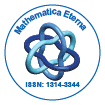# Mathematica EternaOpen Access

ISSN: 1314-3344

## Abstract

### Oscillation of Fractional Nonlinear Difference Equations

S.Lourdu Marian, M. Reni Sagayaraj, A.George Maria Selvam and M.Paul Loganathan

The oscillation criteria for forced nonlinear fractional difference equation of the form ∆αx(t) + f1(t, x(t + α)) =v(t) + f2(t, x(t + α)), t ∈ N0, 0 < α ≤ 1, ∆ α−1x(t)|t=0 =x0, where ∆α denotes the Riemann-Liouville like discrete fractional difference operator of order α is presented.

Top